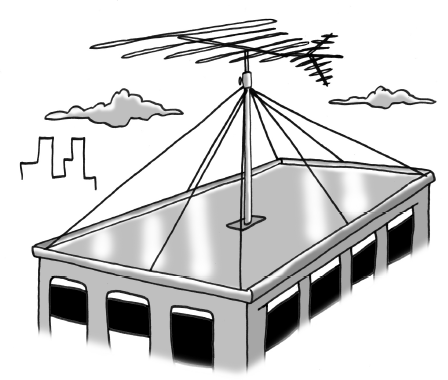### Home > CCA2 > Chapter Ch10 > Lesson 10.1.4 > Problem10-70

10-70.

The Flat Building’s roof is $32$ feet wide and $60$ feet long. An antenna rises $25$ feet above the center of the roof, and wires connect the top of the antenna to each corner of the roof and to the midpoint of each edge, as shown in the picture at right.

1. What was the total length of wire used to connect the antenna to the roof (without counting any extra needed for attaching it)?

First, find the length of the wire connected to the midpoint of the long edge.
You know that the antenna rises $25$ feet ($y$) and the distance from the antenna to the edge is $16$, half of the width ($x$).
Use the Pythagorean Theorem to find the length of the wire.

Use similar strategies to find the lengths of the remaining wires.
The total length is $306.26$ feet of wire.

2. The wires that attach to the corners of the building form an angle with the roof. Find the measure of that angle.

Use inverse tangent to find the angle, using the lengths you derived from part (a).

3. Suppose the height of the antenna is $x$ feet (instead of $25$ feet). Represent the total length of the wires in terms of $x$.

Substitute $x$ for the heights, and add the lengths of the wires.